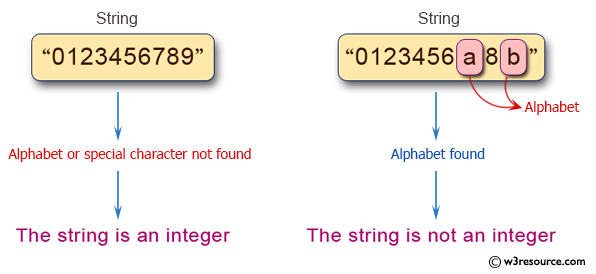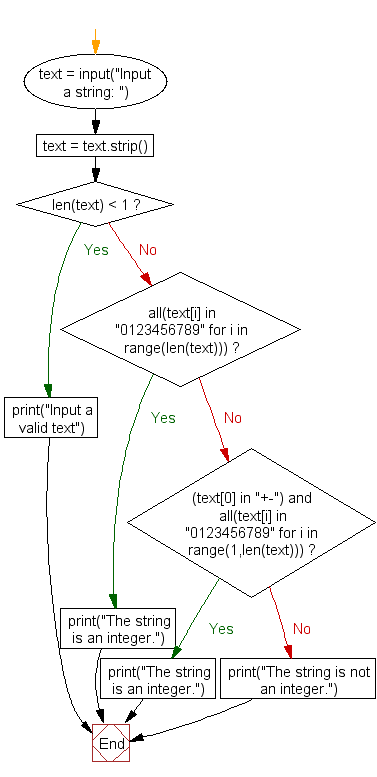﻿ Python Exercise: Check a string represent an integer or not - w3resource# Python Exercise: Check a string represent an integer or not

## Python Conditional: Exercise - 35 with Solution

Write a Python program to check a string represent an integer or not?

Pictorial Presentation:Sample Solution:

Python Code:

``````text = input("Input a string: ")
text = text.strip()
if len(text) < 1:
print("Input a valid text")
else:
if all(text[i] in "0123456789" for i in range(len(text))):
print("The string is an integer.")
elif (text in "+-") and \
all(text[i] in "0123456789" for i in range(1,len(text))):
print("The string is an integer.")
else:
print("The string is not an integer.")
```
```

Sample Output:

```Input a string: python
The string is not an integer.
```

Flowchart :## Visualize Python code execution:

The following tool visualize what the computer is doing step-by-step as it executes the said program:

Python Code Editor:

Have another way to solve this solution? Contribute your code (and comments) through Disqus.

What is the difficulty level of this exercise?

Test your Python skills with w3resource's quiz

﻿

## Python: Tips of the Day

Returns a list with n elements removed from the beginning

Example:

```def tips_take(itr, n = 1):
return itr[:n]
print(tips_take([1, 2, 3], 5))
print(tips_take([1, 2, 3], 0))
```

Output:

```[1, 2, 3]
[]
```